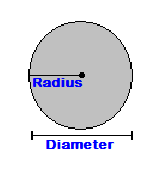# Circle Area Calculator

Find the area of a circle by using this online Circle Calculator.

## Area of a Circle Radius and Diameter

Fill in the radius to find the area of a circle.## How do you find the area of a circle?

Find the area with this circle area formula:
Multiply Pi (3.1416) with the square of the radius (r).
Area = 3.1416 x r squared

The radius can be any measurement of length.
This calculates the area as square units of the length used in the radius.

Example: The area of a circle with a radius(r) of 3 inches is:
Circle Area = 3.1416 x 3 squared

Calculated out this gives an area of 28.2744 Square Inches.